moveit2 The MoveIt Motion Planning Framework for ROS 2.
moveit::core::PlanarJointModel Class Reference

A planar joint. More...

`#include <planar_joint_model.h>`

Inheritance diagram for moveit::core::PlanarJointModel: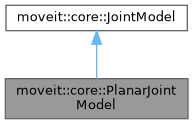[legend]
Collaboration diagram for moveit::core::PlanarJointModel: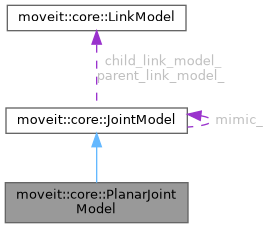[legend]

## Public Types

enum  MotionModel { HOLONOMIC , DIFF_DRIVE }
different types of planar joints we support More...Public Types inherited from moveit::core::JointModel
enum  JointType {
UNKNOWN , REVOLUTE , PRISMATIC , PLANAR ,
FLOATING , FIXED
}
The different types of joints we support. More...

using Bounds = std::vector< VariableBounds >
The datatype for the joint bounds. More...

## Public Member Functions

PlanarJointModel (const std::string &name, size_t joint_index, size_t first_variable_index)

void getVariableDefaultPositions (double *values, const Bounds &other_bounds) const override
Provide a default value for the joint given the joint variable bounds. Most joints will use the default implementation provided in this base class, but the quaternion for example needs a different implementation. Enough memory is assumed to be allocated. More...

void getVariableRandomPositions (random_numbers::RandomNumberGenerator &rng, double *values, const Bounds &other_bounds) const override
Provide random values for the joint variables (within specified bounds). Enough memory is assumed to be allocated. More...

void getVariableRandomPositionsNearBy (random_numbers::RandomNumberGenerator &rng, double *values, const Bounds &other_bounds, const double *near, const double distance) const override
Provide random values for the joint variables (within specified bounds). Enough memory is assumed to be allocated. More...

bool enforcePositionBounds (double *values, const Bounds &other_bounds) const override
Force the specified values to be inside bounds and normalized. Quaternions are normalized, continuous revolute joints are made between -Pi and Pi. Return true if changes were made. More...

bool satisfiesPositionBounds (const double *values, const Bounds &other_bounds, double margin) const override
Check if the set of position values for the variables of this joint are within bounds, up to some margin. More...

void interpolate (const double *from, const double *to, const double t, double *state) const override
Computes the state that lies at time t in [0, 1] on the segment that connects from state to to state. The memory location of state is not required to be different from the memory of either from or to. More...

unsigned int getStateSpaceDimension () const override
Get the dimension of the state space that corresponds to this joint. More...

double getMaximumExtent (const Bounds &other_bounds) const override
Get the extent of the state space (the maximum value distance() can ever report) More...

double distance (const double *values1, const double *values2) const override
Compute the distance between two joint states of the same model (represented by the variable values) More...

void computeTransform (const double *joint_values, Eigen::Isometry3d &transf) const override
Given the joint values for a joint, compute the corresponding transform. The computed transform is guaranteed to be a valid isometry. More...

void computeVariablePositions (const Eigen::Isometry3d &transf, double *joint_values) const override
Given the transform generated by joint, compute the corresponding joint values. Make sure the passed transform is a valid isometry. More...

double getAngularDistanceWeight () const

void setAngularDistanceWeight (double weight)

double getMinTranslationalDistance () const

void setMinTranslationalDistance (double min_translational_distance)

MotionModel getMotionModel () const

void setMotionModel (MotionModel model)

bool normalizeRotation (double *values) constPublic Member Functions inherited from moveit::core::JointModel
JointModel (const std::string &name, size_t joint_index, size_t first_variable_index)
Constructs a joint named name. More...

virtual ~JointModel ()

const std::string & getName () const
Get the name of the joint. More...

JointType getType () const
Get the type of joint. More...

std::string getTypeName () const
Get the type of joint as a string. More...

Get the link that this joint connects to. The robot is assumed to start with a joint, so the root joint will return nullptr here. More...

Get the link that this joint connects to. There will always be such a link. More...

double getDistanceFactor () const
Get the factor that should be applied to the value returned by distance() when that value is used in compound distances. More...

void setDistanceFactor (double factor)
Set the factor that should be applied to the value returned by distance() when that value is used in compound distances. More...

const JointModelgetMimic () const
Get the joint this one is mimicking. More...

double getMimicOffset () const
If mimicking a joint, this is the offset added to that joint's value. More...

double getMimicFactor () const
If mimicking a joint, this is the multiplicative factor for that joint's value. More...

void setMimic (const JointModel *mimic, double factor, double offset)
Mark this joint as mimicking mimic using factor and offset. More...

const std::vector< const JointModel * > & getMimicRequests () const
The joint models whose values would be modified if the value of this joint changed. More...

Notify this joint that there is another joint that mimics it. More...

Get all the link models that descend from this joint, in the kinematic tree. More...

const std::vector< const JointModel * > & getDescendantJointModels () const
Get all the joint models that descend from this joint, in the kinematic tree. More...

const std::vector< const JointModel * > & getNonFixedDescendantJointModels () const
Get all the non-fixed joint models that descend from this joint, in the kinematic tree. More...

bool isPassive () const
Check if this joint is passive. More...

void setPassive (bool flag)

double getMaximumExtent () const

const std::vector< std::string > & getVariableNames () const
Get the names of the variables that make up this joint, in the order they appear in corresponding states. For single DOF joints, this will be just the joint name. For multi-DOF joints these will be the joint name followed by "/", followed by the local names of the variables. More...

const std::vector< std::string > & getLocalVariableNames () const
Get the local names of the variable that make up the joint (suffixes that are attached to joint names to construct the variable names). For single DOF joints, this will be empty. More...

bool hasVariable (const std::string &variable) const
Check if a particular variable is known to this joint. More...

std::size_t getVariableCount () const
Get the number of variables that describe this joint. More...

size_t getFirstVariableIndex () const
Get the index of this joint's first variable within the full robot state. More...

size_t getJointIndex () const
Get the index of this joint within the robot model. More...

size_t getLocalVariableIndex (const std::string &variable) const
Get the index of the variable within this joint. More...

void getVariableDefaultPositions (double *values) const
Provide a default value for the joint given the default joint variable bounds (maintained internally). Most joints will use the default implementation provided in this base class, but the quaternion for example needs a different implementation. Enough memory is assumed to be allocated. More...

void getVariableRandomPositions (random_numbers::RandomNumberGenerator &rng, double *values) const
Provide random values for the joint variables (within default bounds). Enough memory is assumed to be allocated. More...

void getVariableRandomPositionsNearBy (random_numbers::RandomNumberGenerator &rng, double *values, const double *near, const double distance) const
Provide random values for the joint variables (within default bounds). Enough memory is assumed to be allocated. More...

bool satisfiesPositionBounds (const double *values, double margin=0.0) const
Check if the set of values for the variables of this joint are within bounds. More...

bool enforcePositionBounds (double *values) const
Force the specified values to be inside bounds and normalized. Quaternions are normalized, continuous revolute joints are made between -Pi and Pi. Returns true if changes were made. More...

virtual bool harmonizePosition (double *values, const Bounds &other_bounds) const

bool harmonizePosition (double *values) const

bool satisfiesVelocityBounds (const double *values, double margin=0.0) const
Check if the set of velocities for the variables of this joint are within bounds. More...

virtual bool satisfiesVelocityBounds (const double *values, const Bounds &other_bounds, double margin) const
Check if the set of velocities for the variables of this joint are within bounds, up to some margin. More...

bool enforceVelocityBounds (double *values) const
Force the specified velocities to be within bounds. Return true if changes were made. More...

virtual bool enforceVelocityBounds (double *values, const Bounds &other_bounds) const
Force the specified velocities to be inside bounds. Return true if changes were made. More...

bool satisfiesAccelerationBounds (const double *values, double margin=0.0) const
Check if the set of accelerations for the variables of this joint are within bounds. More...

virtual bool satisfiesAccelerationBounds (const double *values, const Bounds &other_bounds, double margin) const
Check if the set of accelerations for the variables of this joint are within bounds, up to some margin. More...

bool satisfiesJerkBounds (const double *values, double margin=0.0) const
Check if the set of jerks for the variables of this joint are within bounds. More...

virtual bool satisfiesJerkBounds (const double *values, const Bounds &other_bounds, double margin) const
Check if the set of jerks for the variables of this joint are within bounds, up to some margin. More...

const VariableBoundsgetVariableBounds (const std::string &variable) const
Get the bounds for a variable. Throw an exception if the variable was not found. More...

const BoundsgetVariableBounds () const
Get the variable bounds for this joint, in the same order as the names returned by getVariableNames() More...

void setVariableBounds (const std::string &variable, const VariableBounds &bounds)
Set the lower and upper bounds for a variable. Throw an exception if the variable was not found. More...

void setVariableBounds (const std::vector< moveit_msgs::msg::JointLimits > &jlim)
Override joint limits loaded from URDF. Unknown variables are ignored. More...

const std::vector< moveit_msgs::msg::JointLimits > & getVariableBoundsMsg () const
Get the joint limits known to this model, as a message. More...Protected Member Functions inherited from moveit::core::JointModel
void computeVariableBoundsMsg ()Protected Attributes inherited from moveit::core::JointModel
JointType type_
The type of joint. More...

std::vector< std::string > local_variable_names_
The local names to use for the variables that make up this joint. More...

std::vector< std::string > variable_names_
The full names to use for the variables that make up this joint. More...

Bounds variable_bounds_
The bounds for each variable (low, high) in the same order as variable_names_. More...

std::vector< moveit_msgs::msg::JointLimits > variable_bounds_msg_

VariableIndexMap variable_index_map_
Map from variable names to the corresponding index in variable_names_ (indexing makes sense within the JointModel only) More...

The link before this joint. More...

The link after this joint. More...

const JointModelmimic_
The joint this one mimics (nullptr for joints that do not mimic) More...

double mimic_factor_
The offset to the mimic joint. More...

double mimic_offset_
The multiplier to the mimic joint. More...

std::vector< const JointModel * > mimic_requests_
The set of joints that should get a value copied to them when this joint changes. More...

Pointers to all the links that will be moved if this joint changes value. More...

std::vector< const JointModel * > descendant_joint_models_
Pointers to all the joints that follow this one in the kinematic tree (including mimic joints) More...

std::vector< const JointModel * > non_fixed_descendant_joint_models_
Pointers to all the joints that follow this one in the kinematic tree, including mimic joints, but excluding fixed joints. More...

bool passive_
Specify whether this joint is marked as passive in the SRDF. More...

double distance_factor_
The factor applied to the distance between two joint states. More...

## Detailed Description

A planar joint.

Definition at line 46 of file planar_joint_model.h.

## ◆ MotionModel

different types of planar joints we support

Enumerator
HOLONOMIC
DIFF_DRIVE

Definition at line 50 of file planar_joint_model.h.

## ◆ PlanarJointModel()

 moveit::core::PlanarJointModel::PlanarJointModel ( const std::string & name, size_t joint_index, size_t first_variable_index )

Definition at line 48 of file planar_joint_model.cpp.

Here is the call graph for this function: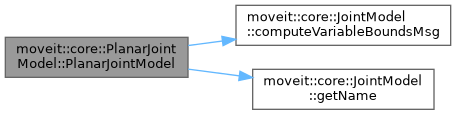## ◆ computeTransform()

 void moveit::core::PlanarJointModel::computeTransform ( const double * joint_values, Eigen::Isometry3d & transf ) const
overridevirtual

Given the joint values for a joint, compute the corresponding transform. The computed transform is guaranteed to be a valid isometry.

Implements moveit::core::JointModel.

Definition at line 345 of file planar_joint_model.cpp.

## ◆ computeVariablePositions()

 void moveit::core::PlanarJointModel::computeVariablePositions ( const Eigen::Isometry3d & transform, double * joint_values ) const
overridevirtual

Given the transform generated by joint, compute the corresponding joint values. Make sure the passed transform is a valid isometry.

Implements moveit::core::JointModel.

Definition at line 351 of file planar_joint_model.cpp.

## ◆ distance()

 double moveit::core::PlanarJointModel::distance ( const double * value1, const double * value2 ) const
overridevirtual

Compute the distance between two joint states of the same model (represented by the variable values)

Implements moveit::core::JointModel.

Definition at line 277 of file planar_joint_model.cpp.

Here is the call graph for this function: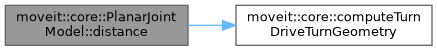Here is the caller graph for this function: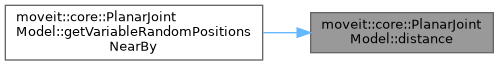## ◆ enforcePositionBounds()

 bool moveit::core::PlanarJointModel::enforcePositionBounds ( double * values, const Bounds & other_bounds ) const
overridevirtual

Force the specified values to be inside bounds and normalized. Quaternions are normalized, continuous revolute joints are made between -Pi and Pi. Return true if changes were made.

Implements moveit::core::JointModel.

Definition at line 326 of file planar_joint_model.cpp.

Here is the call graph for this function: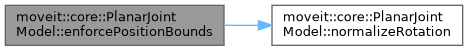## ◆ getAngularDistanceWeight()

 double moveit::core::PlanarJointModel::getAngularDistanceWeight ( ) const
inline

Definition at line 75 of file planar_joint_model.h.

## ◆ getMaximumExtent()

 double moveit::core::PlanarJointModel::getMaximumExtent ( const Bounds & other_bounds ) const
overridevirtual

Get the extent of the state space (the maximum value distance() can ever report)

Implements moveit::core::JointModel.

Definition at line 85 of file planar_joint_model.cpp.

## ◆ getMinTranslationalDistance()

 double moveit::core::PlanarJointModel::getMinTranslationalDistance ( ) const
inline

Definition at line 85 of file planar_joint_model.h.

## ◆ getMotionModel()

 MotionModel moveit::core::PlanarJointModel::getMotionModel ( ) const
inline

Definition at line 95 of file planar_joint_model.h.

## ◆ getStateSpaceDimension()

 unsigned int moveit::core::PlanarJointModel::getStateSpaceDimension ( ) const
overridevirtual

Get the dimension of the state space that corresponds to this joint.

Implements moveit::core::JointModel.

Definition at line 80 of file planar_joint_model.cpp.

## ◆ getVariableDefaultPositions()

 void moveit::core::PlanarJointModel::getVariableDefaultPositions ( double * values, const Bounds & other_bounds ) const
overridevirtual

Provide a default value for the joint given the joint variable bounds. Most joints will use the default implementation provided in this base class, but the quaternion for example needs a different implementation. Enough memory is assumed to be allocated.

Implements moveit::core::JointModel.

Definition at line 92 of file planar_joint_model.cpp.

## ◆ getVariableRandomPositions()

 void moveit::core::PlanarJointModel::getVariableRandomPositions ( random_numbers::RandomNumberGenerator & rng, double * values, const Bounds & other_bounds ) const
overridevirtual

Provide random values for the joint variables (within specified bounds). Enough memory is assumed to be allocated.

Implements moveit::core::JointModel.

Definition at line 109 of file planar_joint_model.cpp.

## ◆ getVariableRandomPositionsNearBy()

 void moveit::core::PlanarJointModel::getVariableRandomPositionsNearBy ( random_numbers::RandomNumberGenerator & rng, double * values, const Bounds & other_bounds, const double * near, const double distance ) const
overridevirtual

Provide random values for the joint variables (within specified bounds). Enough memory is assumed to be allocated.

Implements moveit::core::JointModel.

Definition at line 133 of file planar_joint_model.cpp.

Here is the call graph for this function: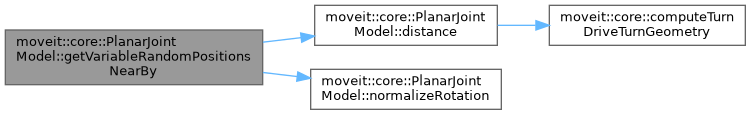## ◆ interpolate()

 void moveit::core::PlanarJointModel::interpolate ( const double * from, const double * to, const double t, double * state ) const
overridevirtual

Computes the state that lies at time t in [0, 1] on the segment that connects from state to to state. The memory location of state is not required to be different from the memory of either from or to.

Implements moveit::core::JointModel.

Definition at line 201 of file planar_joint_model.cpp.

Here is the call graph for this function: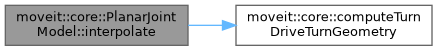## ◆ normalizeRotation()

 bool moveit::core::PlanarJointModel::normalizeRotation ( double * values ) const

Make the yaw component of a state's value vector be in the range [-Pi, Pi]. enforceBounds() also calls this function; Return true if a change is actually made

Definition at line 309 of file planar_joint_model.cpp.

Here is the caller graph for this function: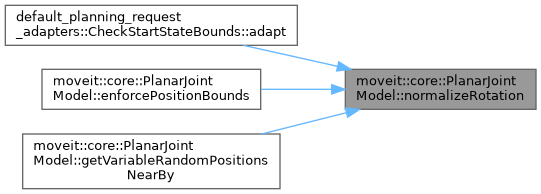## ◆ satisfiesPositionBounds()

 bool moveit::core::PlanarJointModel::satisfiesPositionBounds ( const double * values, const Bounds & other_bounds, double margin ) const
overridevirtual

Check if the set of position values for the variables of this joint are within bounds, up to some margin.

Implements moveit::core::JointModel.

Definition at line 299 of file planar_joint_model.cpp.

## ◆ setAngularDistanceWeight()

 void moveit::core::PlanarJointModel::setAngularDistanceWeight ( double weight )
inline

Definition at line 80 of file planar_joint_model.h.

## ◆ setMinTranslationalDistance()

 void moveit::core::PlanarJointModel::setMinTranslationalDistance ( double min_translational_distance )
inline

Definition at line 90 of file planar_joint_model.h.

## ◆ setMotionModel()

 void moveit::core::PlanarJointModel::setMotionModel ( MotionModel model )
inline

Definition at line 100 of file planar_joint_model.h.

The documentation for this class was generated from the following files: